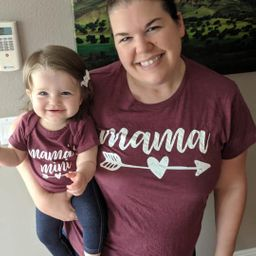Ask your homework questions to teachers and professors, meet other students, and be entered to win $600 or an Xbox Series X 🎉Join our Discord!Numerade Educator ### Problem 58 Hard Difficulty # Find the mean, median, and mode.$$89,19,98,19,108,87$$ ### Answer ## mean$=70,$median$=88$and mode$=19\$

#### Topics

No Related Subtopics

### Discussion

You must be signed in to discuss.

### Video Transcript

by finding them him, which is the average of this data, while at all these numbers together. And we get 420 we'll divide by the number of items in this list, which is six. So that leaves us with 70 as are mean. Our mode is the number that occurs the most amount of times you can see that 19 occurs twice, 19 would be removed and Mosley to find the median. We have to rearrange this in numerical order, so we'll list it from lowest to highest 87 89 98 1 away. The meeting would be the number directly in the middle. Since we haven't even number of items will take the two middle numbers and what Adam together and divide by two you and that leaves us with 88.Other Schools

#### Topics

No Related Subtopics